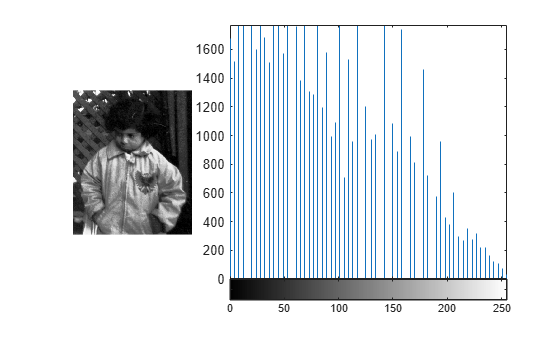# Adjust Image Contrast Using Histogram Equalization

This example shows how to adjust the contrast of a grayscale image using histogram equalization.

Histogram equalization involves transforming the intensity values so that the histogram of the output image approximately matches a specified histogram. By default, the histogram equalization function, `histeq`, tries to match a flat histogram with 64 bins such that the output image has pixel values evenly distributed throughout the range. You can also specify a different target histogram to match a custom contrast.

### Original Image Histogram

Read a grayscale image into the workspace.

`I = imread("pout.tif");`

Display the image and its histogram. The original image has low contrast, with most pixel values in the middle of the intensity range.

```figure subplot(1,3,1) imshow(I) subplot(1,3,2:3) imhist(I)```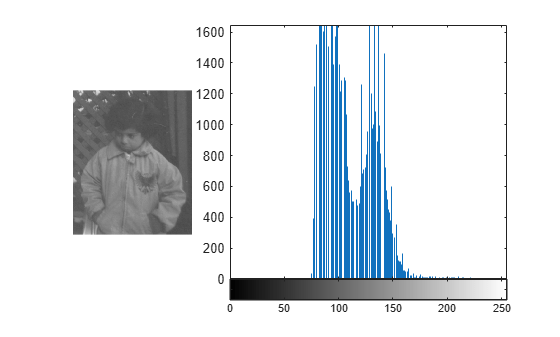### Adjust Contrast Using Default Equalization

Adjust the contrast using histogram equalization. Use the default behavior of the histogram equalization function, `histeq`. The default target histogram is a flat histogram with 64 bins.

`J = histeq(I);`

Display the contrast-adjusted image and its new histogram.

```figure subplot(1,3,1) imshow(J) subplot(1,3,2:3) imhist(J)```### Adjust Contrast, Specifying Number of Bins

Adjust the contrast, specifying a different number of bins. With a small number of bins, there are noticeably fewer gray levels in the contrast-adjusted image.

```nbins =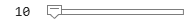10; K = histeq(I,nbins);```

Display the contrast-adjusted image and its new histogram.

```figure subplot(1,3,1) imshow(K) subplot(1,3,2:3) imhist(K)```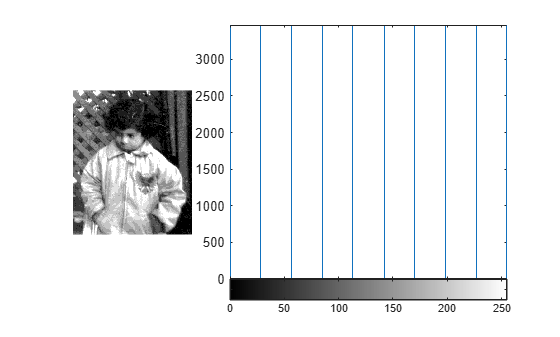### Adjust Contrast, Specifying Target Distribution

Adjust the contrast, specifying a nonflat target distribution. This example demonstrates a linearly decreasing target histogram, which emphasizes small pixel values and causes shadows to appear darker. Display the target histogram.

```target = 256:-4:4; figure bar(4:4:256,target)```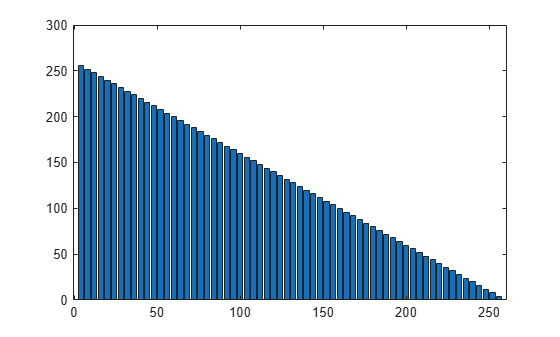Adjust the histogram of the image to approximately match the target histogram.

`L = histeq(I,target);`

Display the contrast-adjusted image and its new histogram.

```figure subplot(1,3,1) imshow(L) subplot(1,3,2:3) imhist(L)```